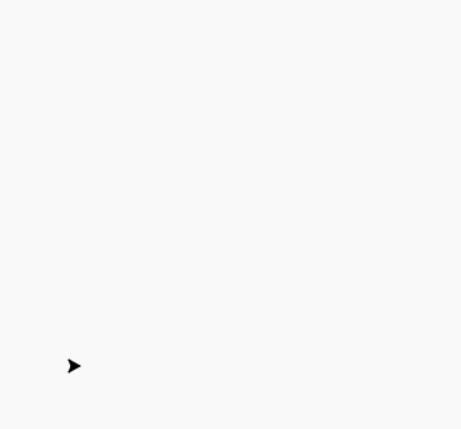Open in App
Not now

# Draw Chess Board Using Turtle in Python

• Last Updated : 01 Aug, 2020

#### Prerequisite:Turtle Programming Basics

Turtle is an inbuilt module in Python. It provides drawing using a screen (cardboard) and turtle (pen). To draw something on the screen, we need to move the turtle (pen). To move turtle, there are some functions i.e forward(), backward(), etc.

For drawing Chess Board following steps are used :

• Import turtle and making an object.
• Set screen size and turtle position.
• Define a method to draw a square
• Call the method 8 times under another loop for 8 times with alternative color.
• Hide the turtle object.

Below is the implementation :

## python3

 `# import turtle package ` `import` `turtle  ` `  `  `# create screen object ` `sc ``=` `turtle.Screen() ` `  `  `# create turtle object ` `pen ``=` `turtle.Turtle() ` `  `  `# method to draw square ` `def` `draw(): ` `  `  `  ``for` `i ``in` `range``(``4``): ` `    ``pen.forward(``30``) ` `    ``pen.left(``90``) ` `  `  `  ``pen.forward(``30``) ` `  `  ` `  `    `  `# Driver Code ` `if` `__name__ ``=``=` `"__main__"` `: ` `     `  `    ``# set screen ` `    ``sc.setup(``600``, ``600``) ` `      `  `    ``# set turtle object speed ` `    ``pen.speed(``100``) ` `      `  `    ``# loops for board ` `    ``for` `i ``in` `range``(``8``): ` `      `  `      ``# not ready to draw ` `      ``pen.up() ` `      `  `      ``# set position for every row ` `      ``pen.setpos(``0``, ``30` `*` `i) ` `      `  `      ``# ready to draw ` `      ``pen.down() ` `      `  `      ``# row ` `      ``for` `j ``in` `range``(``8``): ` `      `  `        ``# conditions for alternative color ` `        ``if` `(i ``+` `j)``%` `2` `=``=` `0``: ` `          ``col ``=``'black'` `      `  `        ``else``: ` `          ``col ``=``'white'` `      `  `        ``# fill with given color ` `        ``pen.fillcolor(col) ` `      `  `        ``# start filling with colour ` `        ``pen.begin_fill() ` `      `  `        ``# call method ` `        ``draw() ` `        ``# stop filling ` `        ``pen.end_fill() ` `      `  `    ``# hide the turtle ` `    ``pen.hideturtle() ` `      `  `    ``# This code is contributed by Deepanshu Rustagi. `

#### Output :My Personal Notes arrow_drop_up
Related Articles